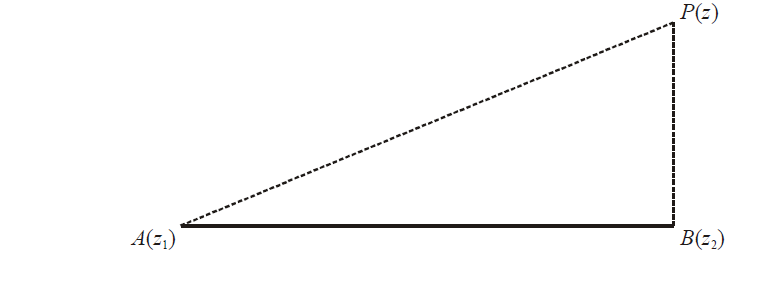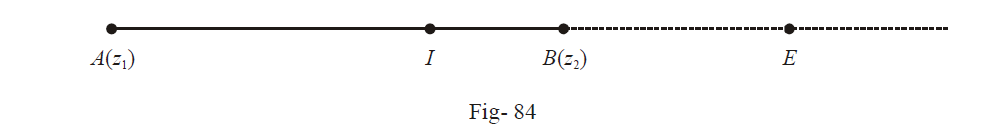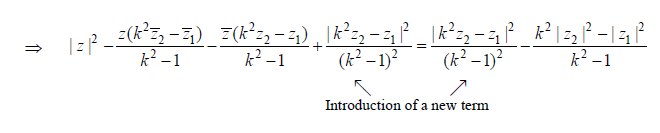# Complex Numbers Set 6

Go back to  'SOLVED EXAMPLES'

Example- 21

Prove that \begin{align}\left| {\frac{{z - {z_1}}}{{z - {z_2}}}} \right| = k\end{align} represents a circle if $$k \ne 1$$  and a line if k = 1.

Solution: For k = 1, the proof is trivial. The given equation reduces to

$|z - {z_1}|\, = \,\,|z - {z_2}|$

which implies that z lies on the perpendicular bisector of the line segment joining Z1 and Z2

Let us now consider the case when $$k \ne 1$$.We need to find the locus of a point P which moves such that

$\frac{{AP}}{{PB}} = K$

Note that one such point also lies on the line segment AB itself which divides AB internally in the ratio k : 1. Also, another such point lies on the extended line of the line segment AB, which will externally divide  AB in the ratio  k : 1.  If k > 1, this external point will lie on the ‘right’ side of B (in the figure above), and if k < 1, this point will lie to the ‘left’ of A.

Assuming k > 1, we let I be the point of internal division and E the point of external division of the segment AB in the ratio k : 1.Thus, \begin{align}\frac{{AI}}{{IB}} = \frac{{AE}}{{EB}} = k.\end{align}

I is given by \begin{align}\frac{{k{z_2} + {z_1}}}{{k + 1}}\end{align}  while E is given by \begin{align}\frac{{k{z_2} - {z_1}}}{{k - 1}}\end{align}

Now, we need the points z which satisfy

\begin{align}&|z - {z_1}|\,\, = \,\,k|z - {z_2}|\\\\&|z{{|}^{2}}+|{{z}_{1}}{{|}^{2}}-z{{\bar{z}}_{1}}-\bar{z}{{z}_{1}}={{k}^{2}}|z{{|}^{2}}+{{k}^{2}}|{{z}_{2}}{{|}^{2}}-{{k}^{2}}z{{\bar{z}}_{2}}-{{k}^{2}}\bar{z}{{z}_{2}}\\\\&\Rightarrow({{k}^{2}}-1)|z{{|}^{2}}-z({{k}^{2}}{{\bar{z}}_{2}}-{{\bar{z}}_{1}})-\bar{z}({{k}^{2}}{{z}_{2}}-{{z}_{1}})+{{k}^{2}}|{{z}_{2}}{{|}^{2}}-|{{z}_{1}}{{|}^{2}}=0\\\\&\Rightarrow |z{{|}^{2}}-z\frac{({{k}^{2}}{{{\bar{z}}}_{2}}-{{{\bar{z}}}_{1}})}{{{k}^{2}}-1}-\bar{z}\frac{({{k}^{2}}{{z}_{2}}-{{z}_{1}})}{{{k}^{2}}-1}+\frac{{{k}^{2}}|{{z}_{2}}{{|}^{2}}-|{{z}_{1}}{{|}^{2}}}{{{k}^{2}}-1}=0\end{align}\begin{align}&\Rightarrow {{\left| z-\frac{{{k}^{2}}{{z}_{2}}-{{z}_{1}}}{{{k}^{2}}-1} \right|}^{2}}=\frac{{{k}^{2}}(|{{z}_{1}}{{|}^{2}}+|{{z}_{2}}{{|}^{2}}-{{z}_{1}}{{{\bar{z}}}_{2}}-{{{\bar{z}}}_{1}}{{z}_{2}})}{{{({{k}^{2}}-1)}^{2}}} =\frac{{{k}^{2}}|{{z}_{1}}-{{z}_{2}}{{|}^{2}}}{{{({{k}^{2}}-1)}^{2}}}\\&\Rightarrow \left| z-\frac{{{k}^{2}}{{z}_{2}}-{{z}_{1}}}{{{k}^{2}}-1} \right|=\frac{k|{{z}_{1}}-{{z}_{2}}|}{{{k}^{2}}-1}\end{align}

This is the equation of a circle with centre at \begin{align}&\frac{{{k^2}{z_2} - {z_1}}}{{{k^2} - 1}}\end{align} and \begin{align}&\frac{{k|{z_1} - {z_2}|}}{{{k^2} - 1}}\end{align} radius. Note that the midpoint of I and E is

$\frac{1}{2}\left( \frac{k{{z}_{2}}+{{z}_{1}}}{k+1}+\frac{k{{z}_{2}}-{{z}_{1}}}{k-1} \right)$

$= \frac{{{k^2}{z_2} - {z_1}}}{{{k^2} - 1}}$

Thus, the center of this circle is actually the mid-point of IE and the radius is \begin{align}\frac{k}{{{k^2} - 1}}\end{align}  times the original line-segment AB.

Example-  22

Find the complex numbers which simultaneously satisfy

\begin{align}&\left| {\frac{{z - 12}}{{z - 8i}}} \right| = \frac{5}{3}\end{align} and \begin{align}&\left| {\frac{{z - 4}}{{z - 8}}} \right| = 1\end{align}

Solution:  Readers who’ve followed the previous example will realise that the first equation is that of a circle while the second is that of a straight line which is the perpendicular bisector of 4 and 8, i.e., from the second equation, we know that z is of the form z = 6 + xi.

Using this in the first equation, we get

$\left| {\frac{{ - 6 + xi}}{{6 + (x - 8)i}}} \right| = \frac{5}{3}$

$\begin{array}{l} \Rightarrow 9({6^2} + {x^2}) = 25\left( {{6^2} + {{(x - 8)}^2}} \right) = 25(100 + {x^2} - 16x)\\ \Rightarrow 16{x^2} - 400x + 2176 = 0\\ \Rightarrow {x^2} - 25x + 136 = 0\\ \Rightarrow (x - 8)(x - 17) = 0\\ \Rightarrow x = 8\,\,\,\,\,{\rm{or}}\,\,\,\,17\end{array}$

Thus, $$z = 6 + 8i\,\,\,{\rm{or}}\,\,\,6 + 17i$$

Example-  23

If the points $${z_1},{z_2},.........{z_n}$$ all lie on the same side of a line passing through the origin, show that the points \begin{align}\frac{1}{{{z_1}}},\frac{1}{{{z_2}}},......,\frac{1}{{{z_n}}}\end{align}  also lie on the same side of another line passing through the origin.

Solution: Let the given line be

$az + \bar a\,\bar z = 0$

All the points $${z_i}$$ lie on the same side of this line, i.e.,

$a{{z}_{i}}+\bar{a}\,{{\bar{z}}_{i}}>0\ \forall \;i \; or \; <0 \forall \;i$

Dividing by $$|{z_i}{|^2} = {z_i}{\bar z_i}$$  on both sides of the inequality,   we obtain

\begin{align}\frac{a}{{{{\bar{z}}}_{i}}}+\frac{{\bar{a}}}{{{z}_{i}}}>0\end{align}  vi  or    <0 vi

\begin{align}\Rightarrow \bar{a}\left( \frac{1}{{{z}_{i}}} \right)+a\overline{\left( \frac{1}{{{z}_{i}}} \right)}>0\end{align}  vi or <0  vi

All the $${z_i}'s$$ lie on the same side of the line $$\bar az + a\bar z = 0$$

Example- 24

Evaluate \begin{align}\sum\limits_{p = 1}^{32} {(3p + 2)} {\left( {\sum\limits_{q = 1}^{10} {\left( {\sin \frac{{2q\pi }}{{11}} - i\cos \frac{{2q\pi }}{{11}}} \right)} } \right)^p}\end{align}

Solution: Although the expression is enormous, the alert reader will quickly realise that this expression can be expressed in terms of the eleventh roots of unity.

\begin{align}{}\left( {\sin \frac{{2q\pi }}{{11}} - i\cos \frac{{2q\pi }}{{11}}} \right) = - i\left( {\cos \frac{{2q\pi }}{{11}} + i\sin \frac{{2q\pi }}{{11}}} \right) \end{align}

$= - i{\alpha ^q}{\rm{\; where\; }}\alpha = \cos \frac{{2\pi }}{{11}} + i\sin \frac{{2\pi }}{{11}}= {e^{i2\pi /11}}$

Thus,

\begin{align}&\sum\limits_{q = 1}^{10} {\left( {\sin \frac{{2q\pi }}{{11}} - i\cos \frac{{2q\pi }}{{11}}} \right)} = \sum\limits_{q = 1}^{10} {( - i{\alpha ^q})} \\ &\qquad\qquad\qquad\qquad\qquad\quad\;\;\;= - i\sum\limits_{q - 1}^{10} {{\alpha ^q}} \\ &\qquad\qquad\qquad\qquad\qquad\quad\;\;\;= - i \times - 1 \left( {1 + \alpha + ...{\alpha ^{10}} = 0} \right)\\ &\qquad\qquad\qquad\qquad\qquad\quad\;\;\;= i\end{align}

The given expression reduces to

$\begin{array}{l}S = \sum\limits_{p = 1}^{32} {(3p + 2){i^p}} \\ = 3\sum\limits_{P = 1}^{32} {p{i^P} + 2\sum\limits_{P = 1}^{32} {{i^P}} } \end{array}$

$$\sum\limits_{P = 1}^{32} {{i^P}}$$ is obviously 0. Therefore

$\begin{array}{l}S = 3\,[(i - 2 - 3i + 4) + (5i - 6 - 7i + 8) + ... - 31\,i + 32]\\\,\,\,\, = 3\,[( - 2i + 2) + ( - 2i + 2) + ...( - 2\,i + 2]\\\,\,\, = 3 \times 8 \times 2(1 - i)\\\,\, = 48\,(1 - i)\end{array}$

Learn from the best math teachers and top your exams

• Live one on one classroom and doubt clearing
• Practice worksheets in and after class for conceptual clarity
• Personalized curriculum to keep up with school